# Word Embedding的简单介绍2019年6月15日07:15:17

6421字阅读21分24秒

## 传统方法介绍

### 1-of-N Encoding

• Have a good day.
• Have a great day.

Have = [1,0,0,0,0]`; a=[0,1,0,0,0]` ; good=[0,0,1,0,0]`; great=[0,0,0,1,0]` ; day=[0,0,0,0,1]`

• 每个word的vector都是独立的, 所以使用1-of-N Encoding没有体现单词与单词之间的关系;
• 如果词汇量很大, 则vector的维度N会很大, 同时造成数据稀疏问题.

### 能否使用Auto-Encoder进行训练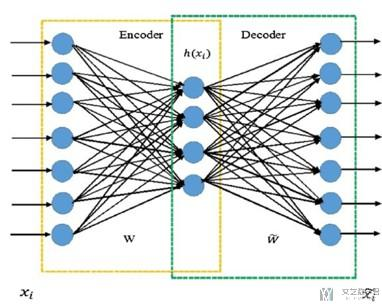• 在使用Auto-Encoder的时候, input和output是相同的.
• 但是由于Word Vector使用1-of-N Encoding, 每一个Vector都是独立的.
• 这样使用Auto-Encoder是学不到东西的.

## Word Embedding介绍

### 想要达到的效果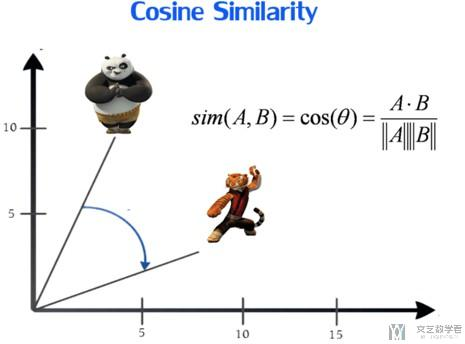### 一些Word Embedding的方式

• 简单Word Embedding
• 给出前一个词汇, 接着预测后一个词汇.
• 进阶方式
• Word2Vec is one of the most popular technique to learn word embeddings using shallow neural network.
• It was developed by Tomas Mikolov in 2013 at Google.
• Continuous bag if word (CBOW)
• Skip-gram

## 基本的Word Embedding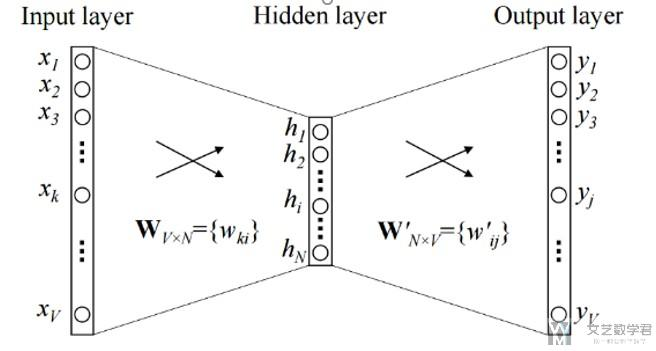• 关于Input : The input or the context word is a one hot encoded vector of size V.
• 关于Hidden layer(最后这个代表一个word的word vector): The hidden layer contains N neurons.
• 关于Output : The output is again a V length vector with the elements being the softmax values.(用来计算误差)

• Wvn is the weight matrix that maps the input x to the hidden layer (V*N dimensional matrix)(其实在这种情况下, Wvn就是最后的word vector, 第一行表示index=1这个单词的vector，因为输入时one hot的)
• W`nv is the weight matrix that maps the hidden layer outputs to the final output layer (N*V dimensional matrix)

### 需要注意:

There is no activation like sigmoid, tanh or ReLU. The only non-linearity is the softmax calculations in the output layer.

• 猫是动物.
• 狗是动物.

## Continuous bag if word(CBOW)## Skip-gram

Use the target word (whose representation we want to generate) to predict the context and in the process。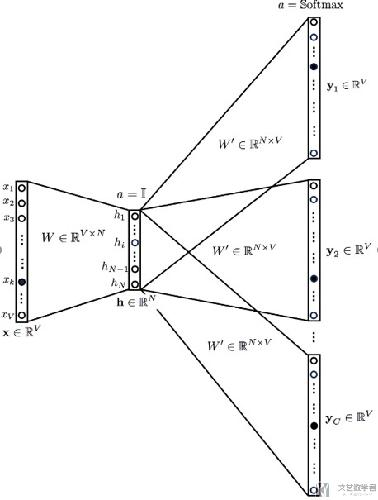## CBOW Model V.S Skip-gram

• According to Mikolov :
• Skip Gram works well with small amount of data and is found to represent rare words well.
• CBOW is faster and has better representations for more frequent words.

### CBOW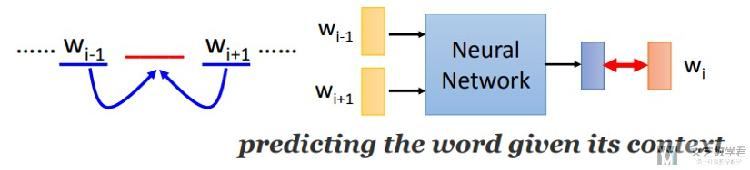### Skip-gram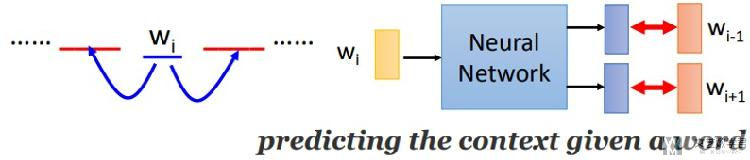## Word Embedding—一些有趣的结果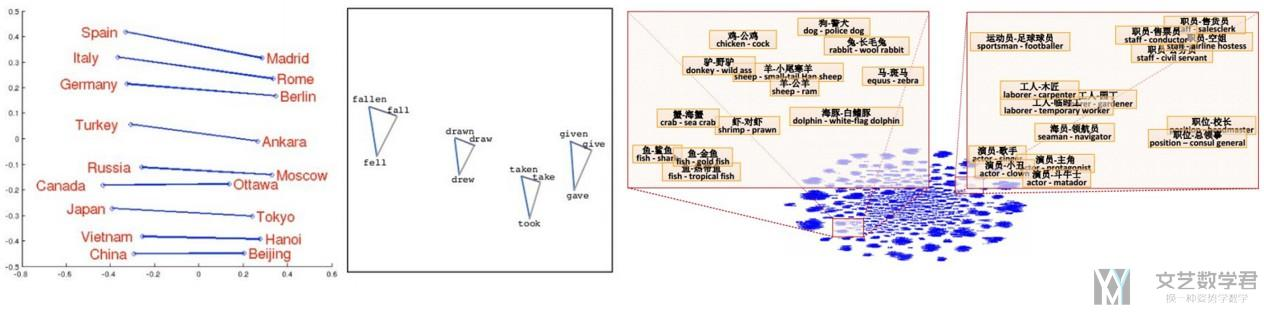## 关于Pytorch中的nn.Embedding功能

Simple lookup table that stores embeddings of a fixed dictionary and size.

This module is often used to store word embeddings and retrieve them using indices. The input to the module is a list of indices, and the output is the corresponding word embeddings.

### 一些解释

nn.Embedding可以看成一个优化过的nn.Linear，我们可以看下面的一个例子。

• 假设共有两个词汇, {'Bruce' : 0,  'Wayne' : 1}
• nn.Embedding的weight是[[0.1,0.2,0.3],[0.2,0.5,0.6]]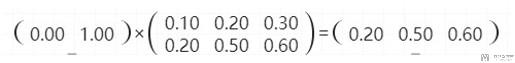### 与nn.Linear的区别

In nn.Embeddings back propagation wouldnt happen on the entire matrix. Back propagation will be done only on the rows of the embedding matrix whose indices are passed.

So, less computation is required in this case.

## Pytorch实现Skip-Gram

### 模型的定义

1. class SkipGram(nn.Module):
2.     def __init__(self, vocab_size, embedding_dim, context_size):
3.         super(SkipGram, self).__init__()
4.         self.vocab_size = vocab_size
5.         self.context_size = context_size
6.         self.embeddings = nn.Embedding(vocab_size, embedding_dim)
7.         self.linear1 = nn.Linear(embedding_dim, 128)
8.         self.linear2 = nn.Linear(128, vocab_size*context_size)
9.     def forward(self, x):
10.         # x的大小为, (batch_size, context_size=1, word_index)
11.         # embedding(x)的大小为, (batch_size, context_size=1, embedding_dim)
12.         # embeds的大小为, (batch_size, context_size*embedding_dim)
13.         batch_size = x.size(0)
14.         embeds = self.embeddings(x).squeeze(1)
15.         output = F.relu(self.linear1(embeds)) # batch_size*128
16.         output = F.log_softmax(self.linear2(output),dim=1).view(batch_size, self.vocab_size, self.context_size) # batch * vocab_size*context_size
17.         output = output.permute(0,2,1)# batch * context_size * vocab_size
18.         return output

### 模型的训练

1. # 模型超参数
2. NUM_EPOCH = 100
3. losses = []
4. loss_function = nn.NLLLoss()
5. model = SkipGram(lang_process.n_words, embedding_dim=30, context_size=2)
7. exp_lr_scheduler = optim.lr_scheduler.StepLR(optimizer, step_size=10, gamma=0.5)
8. # 模型开始训练
9. for epoch in range(NUM_EPOCH):
10.     total_loss = 0
11.     exp_lr_scheduler.step()
12.     for num, (trainData, targetData) in enumerate(train_loader):
13.         # 正向传播
14.         log_probs = model(trainData)
15.         # 两次loss分开加
16.         loss = loss_function(log_probs[:,0,:], targetData[:,0])
17.         loss = loss + loss_function(log_probs[:,1,:], targetData[:,1])
18.         # 反向传播
20.         loss.backward()
21.         optimizer.step()
22.         # Get the Python number from a 1-element Tensor by calling tensor.item()
23.         total_loss += loss.item()
24.     losses.append(total_loss)
25.     if (epoch+1) % 5 == 0:
26.         print('Epoch : {:0>3d}, Loss : {:<6.4f}, Lr : {:<6.7f}'.format(epoch, total_loss, optimizer.param_groups['lr']))

• 微信公众号
• 关注微信公众号
•• QQ群
• 我们的QQ群号
•• 本文由 发表于 2019年6月15日07:15:17
• 转载请务必保留本文链接：https://mathpretty.com/10596.html